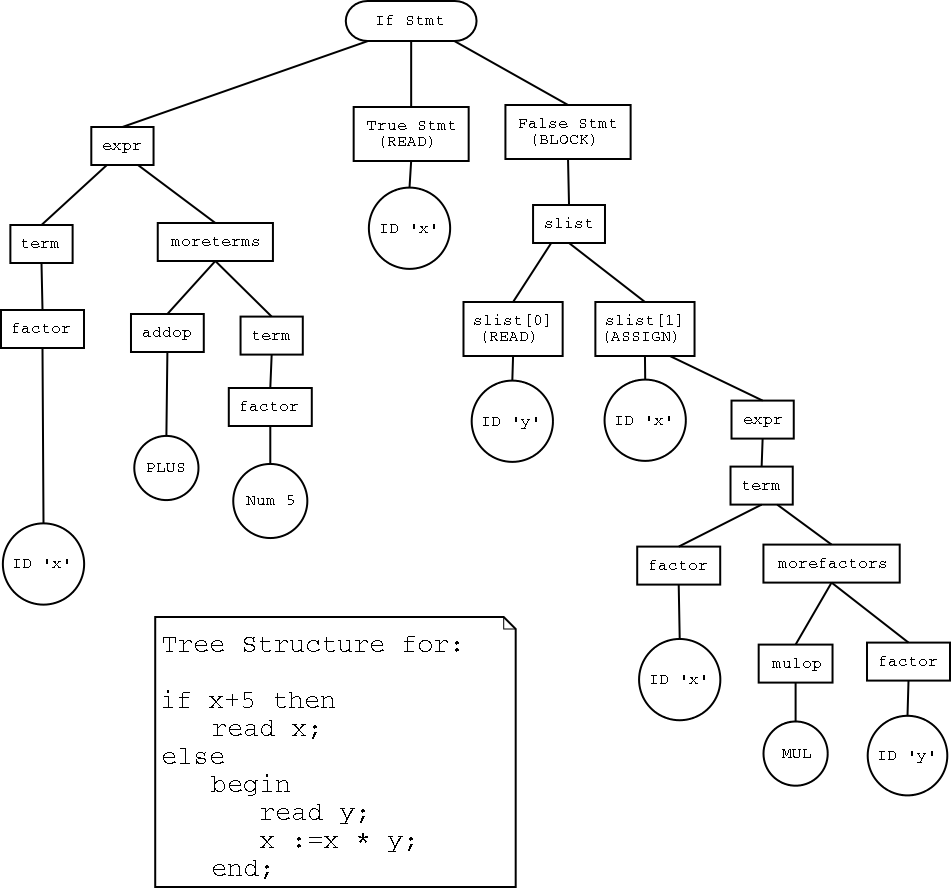# CPSC 411 - Lab Notes - 01-29 (Data Structure)

For recursive descent parsing, we will store the parsed program in a generalized tree, consisting of the semantic elements.

In Python, the most natural way to do this is to define classes that will be the tree elements. The tree itself will be built by the __init__ methods in the respective classes.

The main module of the program will only need to execute a few statements. It will first import the lexer and other modules needed. Then, it will call the lexer with the input code, getting back a list of tokens. We follow that with the following code:

```parsedprog = prog(tokens)
parsedprog.gencode()
```

In the code above, prog is a class whose __init__ accepts the list of tokens and creates an instance of the class. The entire code for the prog class is as follows:

```
class prog:
"""
A class that holds a parsed program. Its only data is a statement."""
def __init__(self,tlist):
self.stmt = stmt(tlist)

def gencode(self):
self.stmt.gencode()

```

(You may use any of the code on this page in your program, but you must give credit to the lab TA's).

Obviously this is quite simple. a more complicated example would be the code to recognize an if statement. Assuming the stmt and expr classes are defined appropriately, we would have the following fragment of the stmt class.

```class stmt:
def __init__(self,tkns):
if tkns.type == 'IF':
self.type = 'IF'
mtoken('IF',tkns)
self.expr = expr(tkns)
mtoken('THEN',tkns)
self.tstmt = stmt(tkns)
mtoken('ELSE',tkns)
self.fstmt = stmt(tkns)
...

def gencode(self):
if self.type == 'IF':
self.expr.gencode()
flab=newlabel()
endlab=newlabel()
print "cJUMP %s"%flab
self.tstmt.gencode()
print "JUMP %s"%endlab
print "%s:"%flab
self.fstmt.gencode()
print "%s:"%endlab

...
```

where the ...stands for code that recognizes other types of statements. mtoken is a module method that matches and returns the next token.

## Graphical representation of an example if statement